# 双流体模型中人工扩散的影响数值分析

1. 西安交通大学动力工程多相流国家重点实验室,陕西 西安 710049
2. 中海油研究总院,北京 100028

# Numerical Analysis of the Effect of Artificial Diffusion Terms on Two-Fluid Model

HAN Peng-fei1, GUO Lie-jin1, LI Qing-ping2, YAO Hai-yuan2, CHENG Bing2

1. State Key Laboratory of Multiphase Flow in Power Engineering, Xi'an Jiaotong University, Xi'an, Shaanxi 710049, China
2. CNOOC Research Institute, Beijing 100028, China

Abstract

When artificial diffusion terms are introduced in two-fluid model, the effects of different artificial diffusion coefficients are studied. For vertical pipe Water Faucet problem, when the artificial diffusion coefficients are gradually increased, nonphysical oscillations can be eliminated. For horizontal pipe flow in ill-posed region, the calculated results without diffusion terms agree with experimental data but the mesh independent solution cannot be obtained. When the coefficients calibrated in Water Faucet problem are adopted, hydrodynamic instability is suppressed to some extent and slug frequency is underestimated. Therefore, the amount of artificial diffusion varies with different flow conditions and should be determined specially.

Keywords： two-fluid model ; artificial diffusion ; slug flow ; numerical simulation

0

HAN Peng-fei, GUO Lie-jin, LI Qing-ping, YAO Hai-yuan, CHENG Bing. Numerical Analysis of the Effect of Artificial Diffusion Terms on Two-Fluid Model[J]. 海洋工程装备与技术, 2015, 2(1): 23-27 https://doi.org/

## 1 控制方程

$∂αlρl∂t$+$∂αlρlul∂x$-$∂∂xρlφal∂αl∂x$=0,(1)

$∂αgρg∂t$+ $∂αgρgug∂x$- $∂∂xρgφag∂αg∂x$=0,(2)

$∂αlρlul∂t$+ $∂αlρlulul∂x$- $∂∂xαlμal∂ul∂x$

=-αl$∂p∂x$lρlgsinθ-αlρlgcosθ $∂hl∂x$-

$τwlSwlA$+ $τiSiA$,(3)

$∂αgρgug∂t$+ $∂αgρgugug∂x$- $∂∂xαgμag∂ug∂x$=-αg$∂p∂x$gρggsinθ-αgρggcosθ $∂hl∂x$- $τwgSwgA$- $τiSiA$,(4)

αlg=1,(5)

τ= $12$$ur$ur,(6)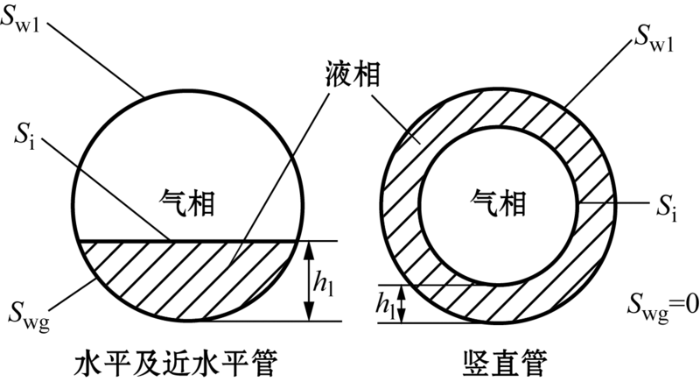Fig.1   Schematic representation of wetted perimeters and two-phase distributions

fi=$16ReiRei<21000.046Rei-0.2Rei≥2100$;(7)

fi=0.005 $1+300hlD$,(8)

D为管道直径.水平管气相与壁面间的摩擦系数同样采用Taitel-Dukler的模型:

fg=$16RegReg<21000.046Reg-0.2Reg≥2100$;(9)

fl=$24RelRel<21000.0262αlRel-0.139Rel≥2100;$(10)

fl=$16RelRel<23000.079Rel-0.25Rel≥2300.$(11)

Rel=$αlρlulDμl$,Reg=$ρgugDgμg$,Rei=$ρgul-ugDgμg$.(12)

## 2 数值方法

$αgρgDρgDt$+$αlρlDρlDt$+∇(αguglul)=0,(13)

## 3 结果及讨论

### 3.1 Water Faucet问题

Water Faucet问题是检验数值计算结果精确度的常用标准方法,其过程如图2所示.其结构为一段12 m长,内径为1 m的竖直管道,上部入口含气率为0.2,气相和液相的速度分别为0和10 m/s,出口压强固定为105 Pa.气相的密度为1.16 kg/m3,液相的密度为1000 kg/m3,整个系统的温度固定为50 ℃.此问题的解析解为

α(x,t)=$αinVinVin2+2g(x-xin)x≤xin+Vint+12gt2αin其他$;(14)

V(x,t)=$Vin2+2g(x-xin)x≤xin+Vint+12gt2Vin+gt其他$. (15)Fig.2   Schematic representation of Water Faucet problem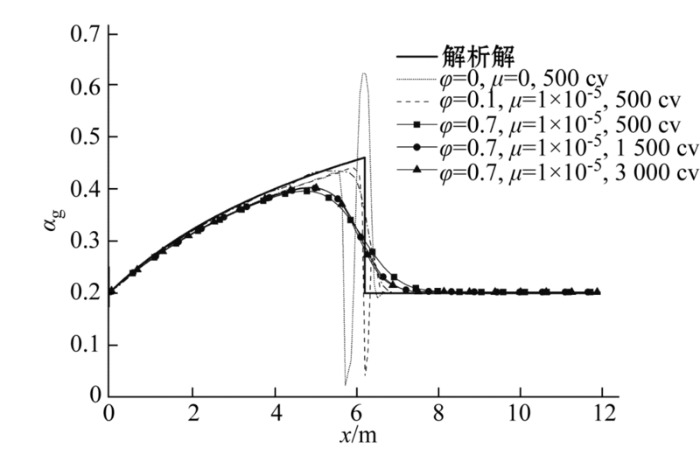Fig.3   Comparison of simulation and analytical results for different diffusion coefficients and mesh densities at t=0.5 s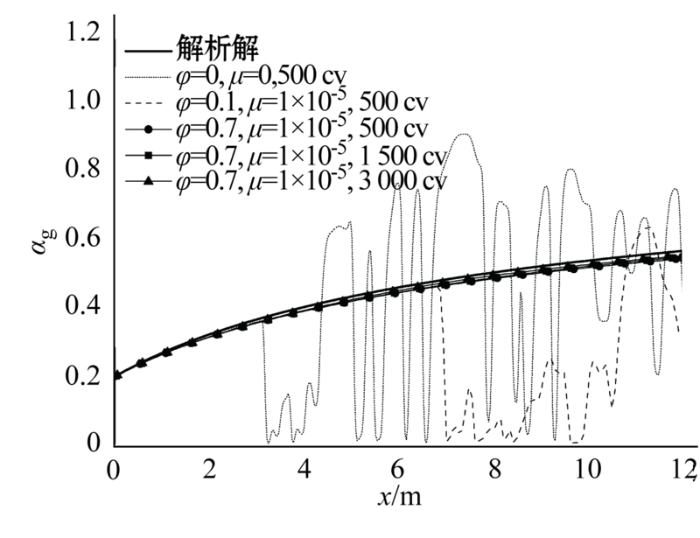Fig.4   Comparison of simulation and analytical results for different diffusion coefficients and mesh densities at t=2.5 s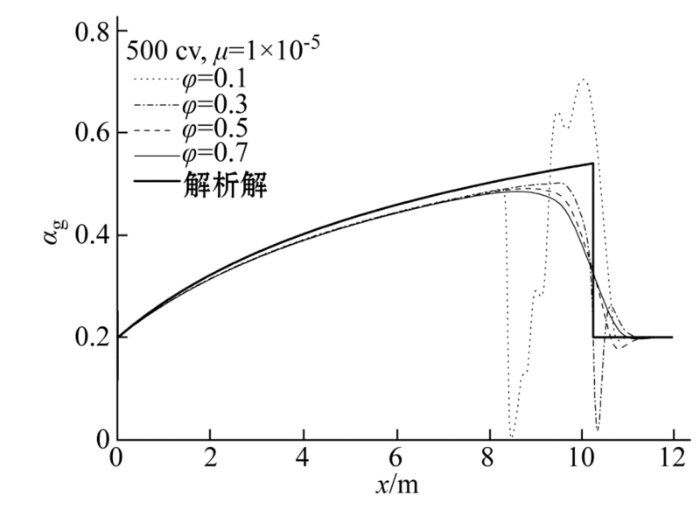Fig.5   Comparison of simulation and analytical results for different diffusion coefficients at t=0.75 s

### 3.2 水平管段塞流

Woods等列举的内径为0.095 m水平管内各种表观气速uSG和表观液速下uSL的段塞流液塞频率如图6所示.本文选取了气液相入口表观速度分别为15.9 m/s和1.2 m/s远离稳定边界的病态区域工况,分别使用较粗网格不加入人工扩散和人工扩散系数φ=0.7,μ=1×10-5进行计算.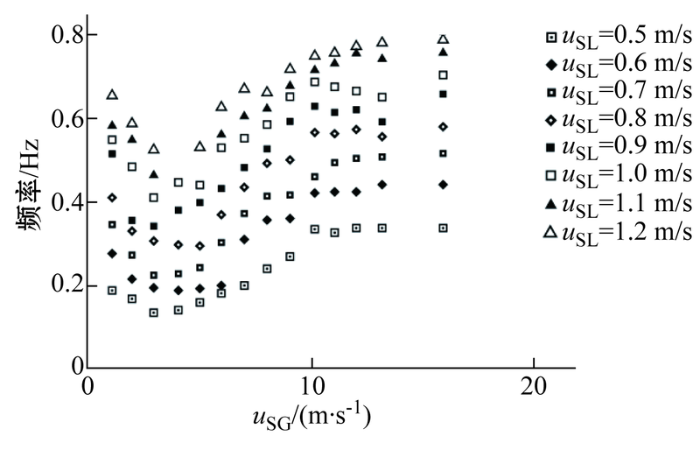Fig.6   Experimental data for slug frequency presented by Woods et al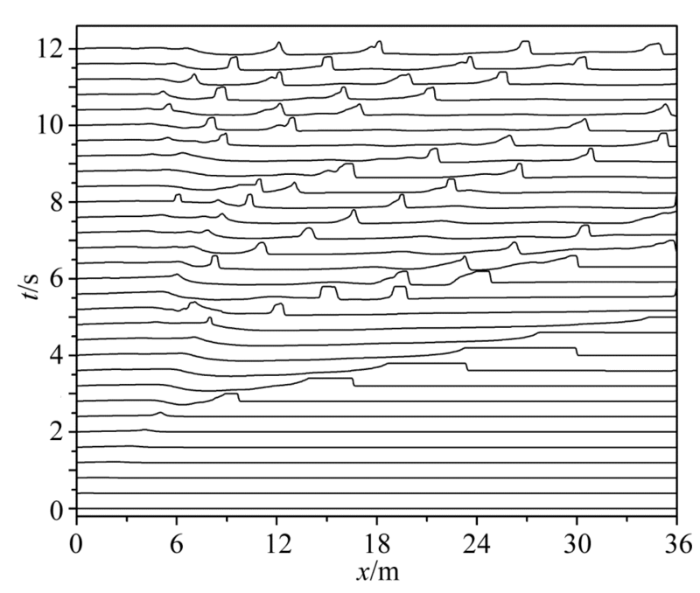Fig.7   Time trace of simulated non-dimensional liquid film height along the pipe (φ=μ=0)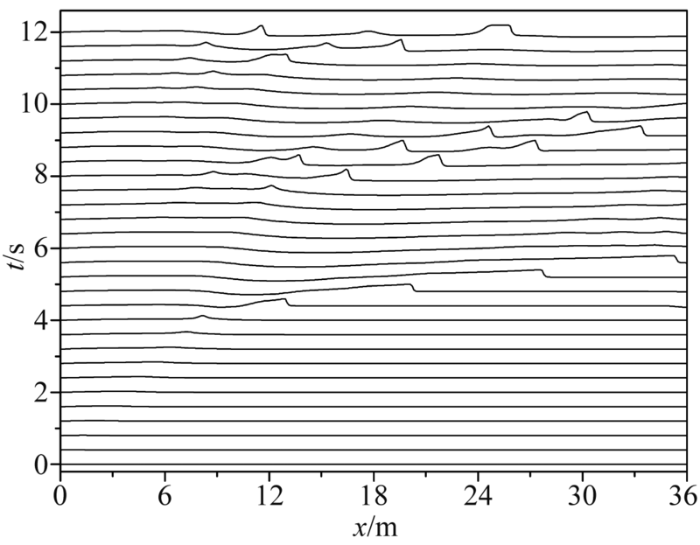Fig.8   Time trace of simulated non-dimensional liquid film height along the pipe(φ=0.7,μ=1×10-5)

## 4 结 语

The authors have declared that no competing interests exist.

## 参考文献 原文顺序 文献年度倒序 文中引用次数倒序 被引期刊影响因子

  Lin P Y, Hanratty T J.Prediction of the initiation of slugs with linear stability theory[J]. International Journal of Multiphase Flow, 1986, 12(1): 79. This paper explores the application of linear stability theory to explain the onset of slugging. It is shown that the inviscid Kelvin-Helmholtz theory correctly predicts stability of a stratified flow only for very large liquid viscosities. In general, however, inviscid theory is in error because it ignores the destabilizing effect of liquid inertia. Good agreement is noted between the linear stability analysis and observations of the initiation of slugs in 2.54- and 9.53-cm horizontal pipes at superficial gas velocities less than 3.3 m/s.  Issa R I, Kempf M H W. Simulation of slug flow in horizontal and nearly horizontal pipes with the two-fluid model[J]. International Journal of Multiphase Flow, 2003, 29(1): 69.

Abstract

A mechanistic approach to the prediction of hydrodynamic slug initiation, growth and subsequent development into continuous slug flow in pipelines is presented. The approach is based on the numerical solution of the one-dimensional transient two-fluid model equations. The advantage of this approach is that the flow field is allowed to develop naturally from any given initial conditions as part of the transient calculation; the slugs evolve automatically as a product of the computed flow development. The need for the many phenomenological models for flow regime transition, formation of slugs and their dynamics can thus be minimized.

It is shown that when the two-fluid model is invoked within the confines of the conditions under which it is mathematically well-posed, it is capable of capturing the growth of instabilities in stratified flow leading to the generation of slugs. The computed rates of growth of such instabilities compare well with the values obtained from Kelvin–Helmholtz analyses. Simulations are then carried out for a large number of pipe configurations and flow conditions that lead to slug flow. These include horizontal, inclined and V-section pipes. The results of computations for slug characteristics are compared with data obtained from the literature and it is found that the agreement is remarkable given the simplicity of the one-dimensional model.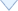〈〉